• MCP神经元和罗森布拉特筏值感知机的理念：  通过模拟的方式还原大脑中单个神经元的工作方式（是否被激活）   罗森布拉特筏值感知机最初的规则：  将权重初始化为0或者一个极小的数  迭代所有训练样本x  ...
本文代码推荐使用Jupyter notebook跑，这样得到的结果更为直观。
感知机
MCP神经元和罗森布拉特筏值感知机的理念：
通过模拟的方式还原大脑中单个神经元的工作方式（是否被激活）

罗森布拉特筏值感知机最初的规则：
将权重初始化为0或者一个极小的数
迭代所有训练样本x
计算输出值y
更新权重

把分类问题看作一个二值分类任务，一般记为1和-1。
定义一个激励函数，激励函数以特定的输入值x与相应的权值向量w的线性组合作为输入。如果激励函数的输出值大于预设的筏值θ，将其分到1类，否则分到-1类。

感知机算法中，激励函数是一个简单的分段函数。

权重w的更新方式为：wj = wj +用于更新wj的值
更新权重wj的值 = n（yi - ^yi）xj
n为学习速率，介于0-1之间的常数
^yi为预测得到的类标
权重值是同时更新的

感知机收敛的前提：两个类别必须是线性可分的，且学习速率足够小
感知机不收敛会不断的更新权值，解决办法：
设置迭代次数最大值，强制停止感知机
设置允许错误分类样本的筏值

线性可分与非线性可分：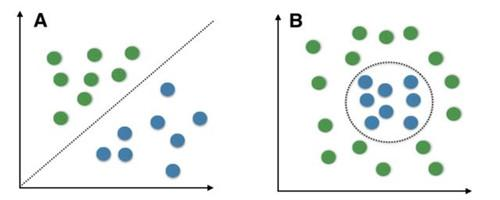python实现感知机算法：

import numpy as np
class Perceptron(object):
"""感知器分类器

参数
------------
eta : float
学习速率 (between 0.0 and 1.0)
n_iter : int
通过训练数据集

属性
-----------
w_ : 1d-array
加权拟合后.
errors_ : list
每一个时代的错误分类数目。

"""
def __init__(self, eta=0.01, n_iter=10):
self.eta = eta
self.n_iter = n_iter

def fit(self, X, y):
"""训练数据。
参数
----------
X : {array-like}, shape = [n_samples, n_features]
培训向量,n_samples样品和n_features的数量特征的数量。
y : array-like, shape = [n_samples]
目标的值。

Returns
-------
self : object

"""
self.w_ = np.zeros(1 + X.shape)
self.errors_ = []

for _ in range(self.n_iter):
errors = 0
for xi, target in zip(X, y):
update = self.eta * (target - self.predict(xi))
self.w_[1:] += update * xi
self.w_ += update
errors += int(update != 0.0)
self.errors_.append(errors)
return self

def net_input(self, X):
"""计算净输入"""
# 计算向量点积
return np.dot(X, self.w_[1:]) + self.w_

def predict(self, X):
"""在单位步骤后返回类标签。计算和预测类标"""
return np.where(self.net_input(X) >= 0.0, 1, -1)


Numpy用于算术运算向量化，C语言实现的python科学计算库。
向量化的好处：一个算术运算操作会自动应用到数组中的所有元素上

基于鸢尾花数据集训练了感知机模型：
# 1、获取数据集
import pandas as pd
print(df.tail())

#/2、抽取100个类标，50个setosa和50个versicolor，显示二维散点图。
import matplotlib.pyplot as plt
import numpy as np

#选择setosa和杂色的
y = df.iloc[0:100, 4].values
# 1代表versicolor，-1代表setosa
y = np.where(y == 'Iris-setosa', -1, 1)

# 提取萼片长度和花瓣长度。
X = df.iloc[0:100, [0, 2]].values

# plot data
plt.scatter(X[:50, 0], X[:50, 1],
color='red', marker='o', label='setosa')
plt.scatter(X[50:100, 0], X[50:100, 1],
color='blue', marker='x', label='versicolor')

plt.xlabel('petal length [cm]')
plt.ylabel('sepal length [cm]')
plt.legend(loc='upper left')

plt.tight_layout()
# plt.savefig('./iris_1.png', dpi=300)
plt.show()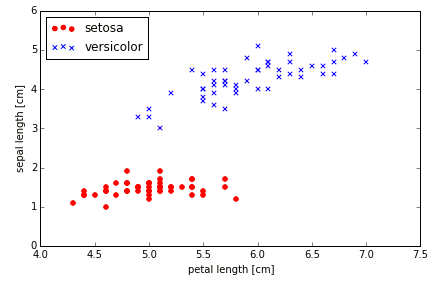# 3、取数据集训练感知机模型，显示错误分类数量和迭代次数的折线图
ppn = Perceptron(eta=0.1, n_iter=10)

ppn.fit(X, y)

plt.plot(range(1, len(ppn.errors_) + 1), ppn.errors_, marker='o')
plt.xlabel('Epochs')
plt.ylabel('Number of misclassifications')

plt.tight_layout()
# plt.savefig('./perceptron_1.png', dpi=300)
plt.show()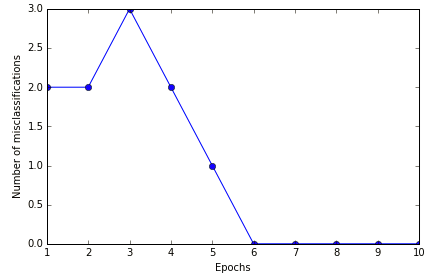# 4、显示二维数据集决策边界
from matplotlib.colors import ListedColormap

def plot_decision_regions(X, y, classifier, resolution=0.02):

# setup marker generator and color map
markers = ('s', 'x', 'o', '^', 'v')
colors = ('red', 'blue', 'lightgreen', 'gray', 'cyan')
cmap = ListedColormap(colors[:len(np.unique(y))])

# plot the decision surface
x1_min, x1_max = X[:, 0].min() - 1, X[:, 0].max() + 1
x2_min, x2_max = X[:, 1].min() - 1, X[:, 1].max() + 1
xx1, xx2 = np.meshgrid(np.arange(x1_min, x1_max, resolution),
np.arange(x2_min, x2_max, resolution))
Z = classifier.predict(np.array([xx1.ravel(), xx2.ravel()]).T)
Z = Z.reshape(xx1.shape)
plt.contourf(xx1, xx2, Z, alpha=0.4, cmap=cmap)
plt.xlim(xx1.min(), xx1.max())
plt.ylim(xx2.min(), xx2.max())

# plot class samples
for idx, cl in enumerate(np.unique(y)):
plt.scatter(x=X[y == cl, 0], y=X[y == cl, 1],
alpha=0.8, c=cmap(idx),
marker=markers[idx], label=cl)

plot_decision_regions(X, y, classifier=ppn)
plt.xlabel('sepal length [cm]')
plt.ylabel('petal length [cm]')
plt.legend(loc='upper left')

plt.tight_layout()
# plt.savefig('./perceptron_2.png', dpi=300)
plt.show()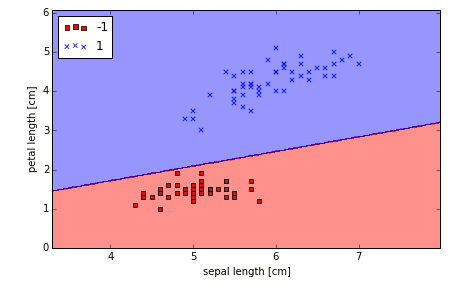自适应线性神经元：
单层神经网络：自适应线性神经元

线性激励函数在权重更新时，使用量化器对类标进行预测，量化器与单位跃阶函数类似。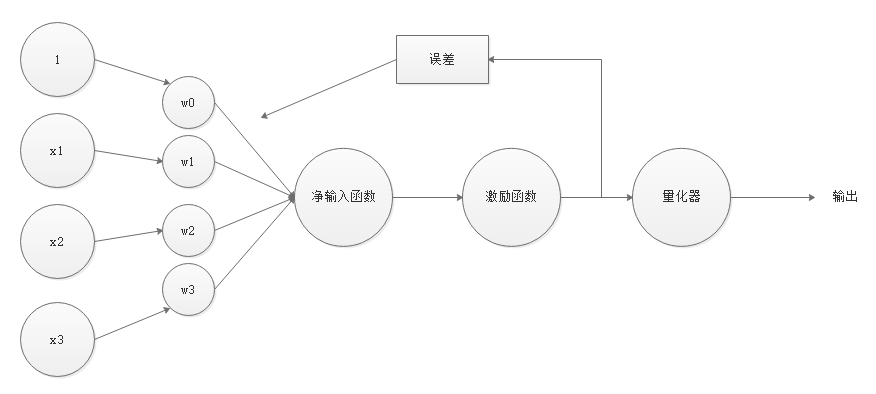使用线性激励函数的连续型输出值，而不是而类别分类类标来计算模型的误差以及更新权重。

使用梯度下降最小化代价函数
监督学习算法的一个核心组成：在学习阶段定义一个待优化的目标函数。
这个目标函数通常是需要做最小化处理的代价函数。
可以将代价函数定义为通过模型得到输出与实际类标之间的误差平方和。
连续型激励函数主要优点是可导，而且是一个凸函数，这样可以通过简单高效的梯度下降算法来得到权重。

梯度下降图：

为了计算代价函数的梯度，计算代价函数相对于每个权重的偏导。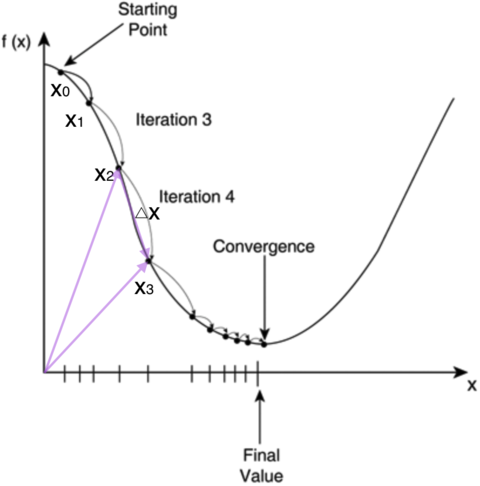python实现自适应线性神经元：

class AdalineGD(object):

Parameters
------------
eta : float
Learning rate (between 0.0 and 1.0)
n_iter : int
Passes over the training dataset.

Attributes
-----------
w_ : 1d-array
Weights after fitting.
errors_ : list
Number of misclassifications in every epoch.

"""
def __init__(self, eta=0.01, n_iter=50):
self.eta = eta
self.n_iter = n_iter

def fit(self, X, y):
""" Fit training data.

Parameters
----------
X : {array-like}, shape = [n_samples, n_features]
Training vectors, where n_samples is the number of samples and
n_features is the number of features.
y : array-like, shape = [n_samples]
Target values.

Returns
-------
self : object

"""
self.w_ = np.zeros(1 + X.shape)
self.cost_ = []

for i in range(self.n_iter):
output = self.net_input(X)
errors = (y - output)
self.w_[1:] += self.eta * X.T.dot(errors)
self.w_ += self.eta * errors.sum()
cost = (errors**2).sum() / 2.0
self.cost_.append(cost)
return self

def net_input(self, X):
"""Calculate net input"""
return np.dot(X, self.w_[1:]) + self.w_

def activation(self, X):
"""Compute linear activation"""
return self.net_input(X)

def predict(self, X):
"""Return class label after unit step"""
return np.where(self.activation(X) >= 0.0, 1, -1)

# 标准化的特征
X_std = np.copy(X)
X_std[:,0] = (X[:,0] - X[:,0].mean()) / X[:,0].std()
X_std[:,1] = (X[:,1] - X[:,1].mean()) / X[:,1].std()

plt.xlabel('sepal length [standardized]')
plt.ylabel('petal length [standardized]')
plt.legend(loc='upper left')
plt.tight_layout()
plt.show()

plt.xlabel('Epochs')
plt.ylabel('Sum-squared-error')

plt.tight_layout()
plt.show()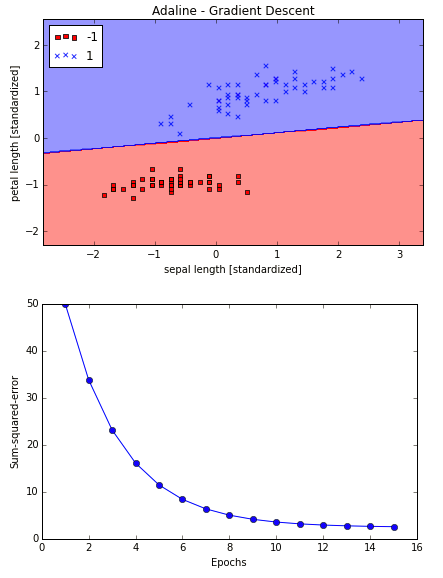# 绘制两种不同学习速率下，代价函数与迭代次数的图像。
fig, ax = plt.subplots(nrows=1, ncols=2, figsize=(8, 4))

ax.set_xlabel('Epochs')
ax.set_ylabel('log(Sum-squared-error)')

ax.set_xlabel('Epochs')
ax.set_ylabel('Sum-squared-error')

plt.tight_layout()
plt.show()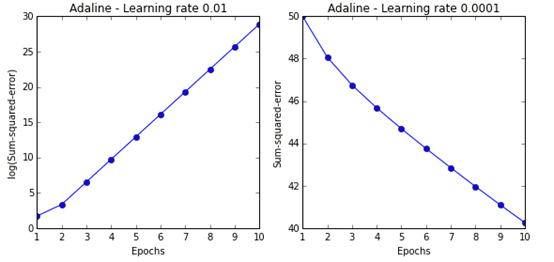第一张图的学习速率过大跳过全局最优解，导致误差随迭代次数上升而上升。

学习率过大的效果图：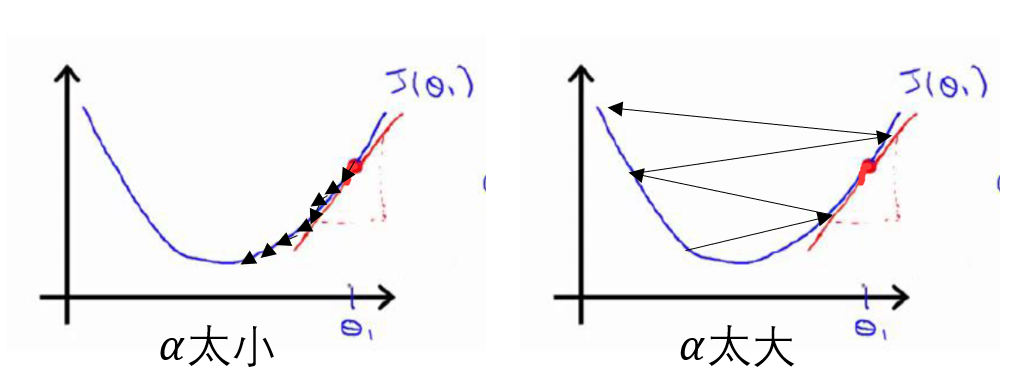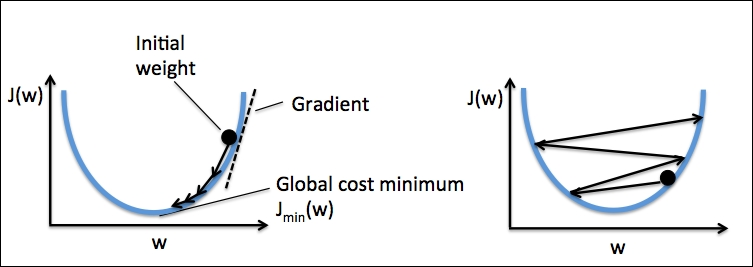随机梯度下降：

大数据集使用梯度下降成本高昂，随机梯度下降能解决这个问题。
随机梯度下降也称为迭代梯度下降或者在线梯度下降。
随机梯度下降更容易跳出最小范围的局部最优解，为了得到更加准确的结果，让数据随机提供给算法是非常重要的，防止每次迭代打乱训练集以防进入死循环。

随机梯度下降的学习速率随着时间变化的：n = c1/(迭代次数+c2)，c1 c2为常数。

随机梯度下降可将用于在线学习，有新的数据输入时模型会被实时训练。

随机梯度下降：
大数据集使用梯度下降成本高昂，随机梯度下降能解决这个问题。
随机梯度下降也称为迭代梯度下降或者在线梯度下降。
随机梯度下降更容易跳出最小范围的局部最优解，为了得到更加准确的结果，让数据随机提供给算法是非常重要的，防止每次迭代打乱训练集以防进入死循环。

随机梯度下降的学习速率随着时间变化的：n = c1/(迭代次数+c2)，c1 c2为常数。

随机梯度下降可将用于在线学习，有新的数据输入时模型会被实时训练。

python实现随机梯度下降：
from numpy.random import seed

Parameters
------------
eta : float
Learning rate (between 0.0 and 1.0)
n_iter : int
Passes over the training dataset.

Attributes
-----------
w_ : 1d-array
Weights after fitting.
errors_ : list
每一个迭代的错误分类数目。
shuffle : bool (default: True)
如果要防止循环的话，每个阶段都要训练数据。
random_state : int (default: None)
设置随机状态，以调整和初始化权重。

"""
def __init__(self, eta=0.01, n_iter=10, shuffle=True, random_state=None):
self.eta = eta
self.n_iter = n_iter
self.w_initialized = False
self.shuffle = shuffle
if random_state:
seed(random_state)

def fit(self, X, y):
""" 训练数据。

Parameters
----------
X : {array-like}, shape = [n_samples, n_features]
Training vectors, where n_samples is the number of samples and
n_features is the number of features.
y : array-like, shape = [n_samples]
Target values.

Returns
-------
self : object

"""
self._initialize_weights(X.shape)
self.cost_ = []
for i in range(self.n_iter):
if self.shuffle:
X, y = self._shuffle(X, y)
cost = []
for xi, target in zip(X, y):
cost.append(self._update_weights(xi, target))
avg_cost = sum(cost)/len(y)
self.cost_.append(avg_cost)
return self

def partial_fit(self, X, y):
"""在不重新初始化权重的情况下调整训练数据。"""
if not self.w_initialized:
self._initialize_weights(X.shape)
if y.ravel().shape > 1:
for xi, target in zip(X, y):
self._update_weights(xi, target)
else:
self._update_weights(X, y)
return self

def _shuffle(self, X, y):
""打乱训练数据"""
r = np.random.permutation(len(y))
return X[r], y[r]

def _initialize_weights(self, m):
"""初始化权重为零"""
self.w_ = np.zeros(1 + m)
self.w_initialized = True

def _update_weights(self, xi, target):
output = self.net_input(xi)
error = (target - output)
self.w_[1:] += self.eta * xi.dot(error)
self.w_ += self.eta * error
cost = 0.5 * error**2
return cost

def net_input(self, X):
"""计算净输入"""
return np.dot(X, self.w_[1:]) + self.w_

def activation(self, X):
"""计算线性激活"""
return self.net_input(X)

def predict(self, X):
"""在单位步骤后返回类标签。"""
return np.where(self.activation(X) >= 0.0, 1, -1)

plt.xlabel('sepal length [standardized]')
plt.ylabel('petal length [standardized]')
plt.legend(loc='upper left')

plt.tight_layout()
plt.show()

plt.xlabel('Epochs')
plt.ylabel('Average Cost')

plt.tight_layout()
plt.show()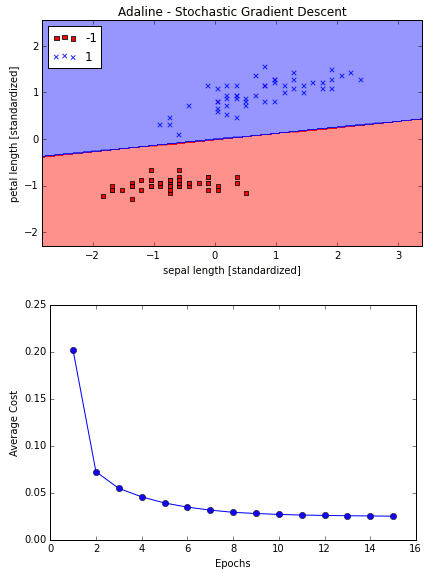展开全文机器学习 梯度下降法 python
• 轴突树突是神经元的突起，在神经元之间传递的是电信号，神经元的功能是接受信号并对其作出迅速反应、传导兴奋等。 当神经元之间相互连接，当某一个神经元处于兴奋 状态时，其相连神经元的电位将发生改变，若神经...
神经元
人工神经网络与生物神经网络相似，都是由大量神经元组成，其中神经元又被称之为神经细胞，都具有细胞体、胞体、树突以及轴突等。
轴突和树突是神经元的突起，在神经元之间传递的是电信号，神经元的功能是接受信号并对其作出迅速反应、传导兴奋等。
当神经元之间相互连接，当某一个神经元处于兴奋 状态时，其相连神经元的电位将发生改变，若神经元电位改变超过一定的数值时，则相连的神经元被激活并处于兴奋状态，向下一级连接的神经元继续传递电位改变信息。信息从一个神经元一电传导的方式跨过之间的链接，传给另一个神经元，最终使肌肉收缩或分泌液体。
神经元就是一个多个输入、输出的信息处理单元，并且对信息的处理是非线性的。
1943年McCulloch 和Pitts提出MP模型，由固定权值和权重组成，是一种基于阈值逻辑算法的神经网络计算模型
在MP模型中，某个神经元接受来自其余多个神经元的传递信息号，多个输入与对应连接权重相乘后并对其求合，再与神经元预设的阈值进行比较，最后通过激活函数产生神经元输出。每个神经元具有空间整合特性和阈值特性。
感知机
感知机是由Frank Rosenblatt在1957年提出的模型，与MP类似。
一般感知机指单层人工神经网络，以区别与多层感知机，其主要结构如下图所示：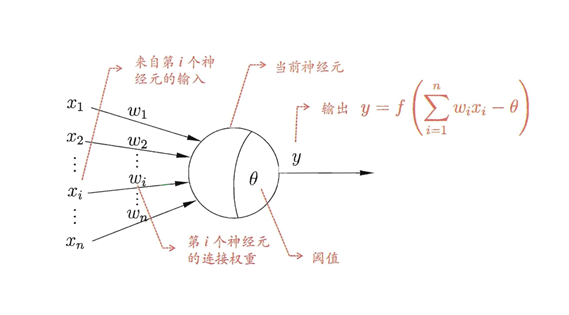假设有一个n维输入的单层感知机，x1到xn为n维输入向量的各个分量，w1到wn为各个输入分量连接到感知机的权重，θ为阈值，f为激活函数，y为标量输出。理想的激活函数f(.) 通常为阶跃函数或sigmoid函数。感知机的输出是输入向量x与权重向量的W求得内积 后，经激活函数f所得到的标量 ：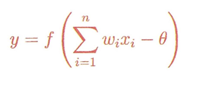为了得到更好的拟合输出结果，权重W的初始值一般为随机值。通过计算得到的输出值，然后将实际输出值和理论值输出值做差，以此来调整每一个输出值的权值。学校规则是用来计算新的权值矩阵W及新的偏差B的算法。 
展开全文深度学习
• 下面的图是1层有多个神经元，但是只有一层，没有达到深度学习的“要求”。...下图是两层，相对比于线性回归的单层多个神经元和没有层只有一个神经元的模型，深了一些。所以下图的模型就叫做多层感知器。 ...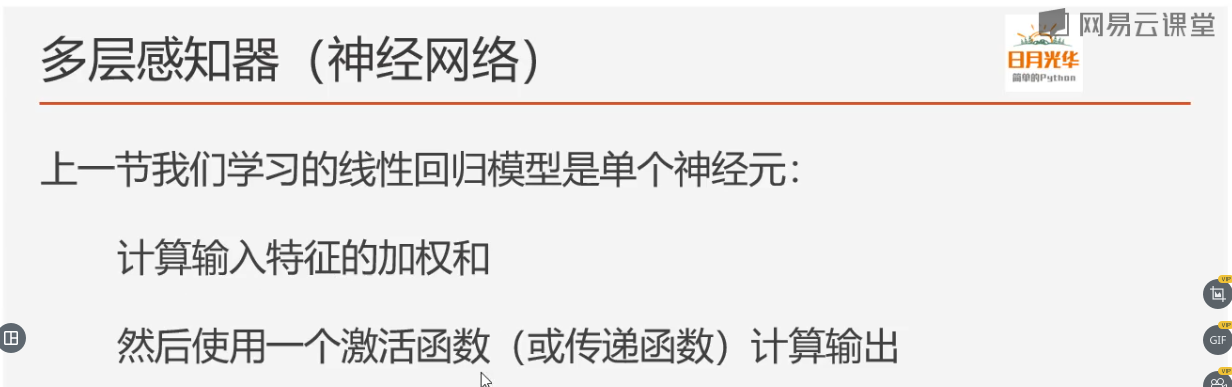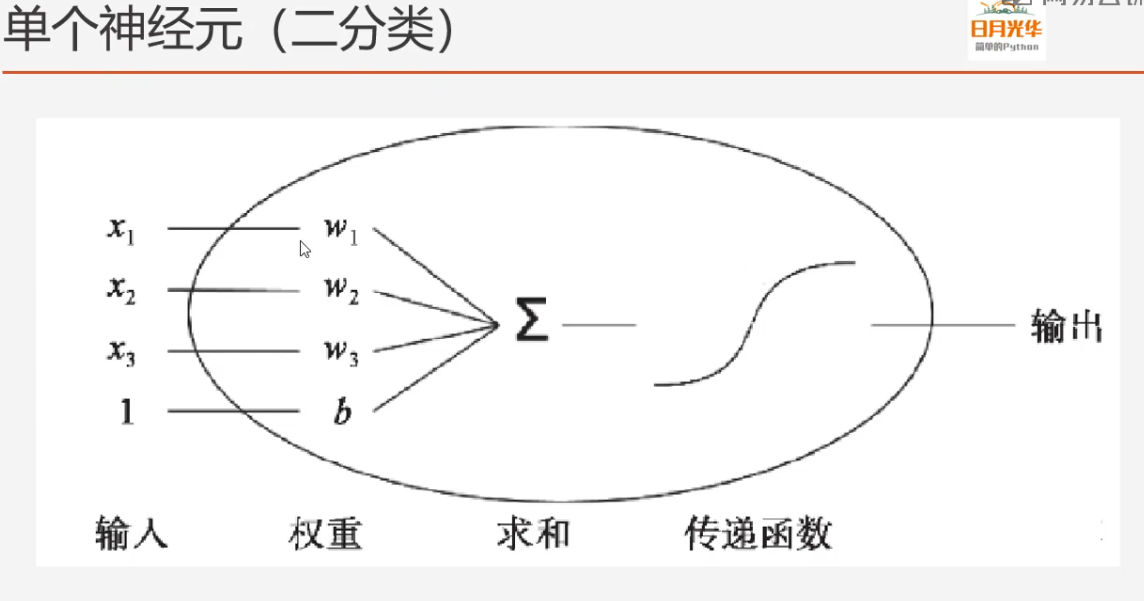下面的图是1层有多个神经元，但是只有一层，没有达到深度学习的“要求”。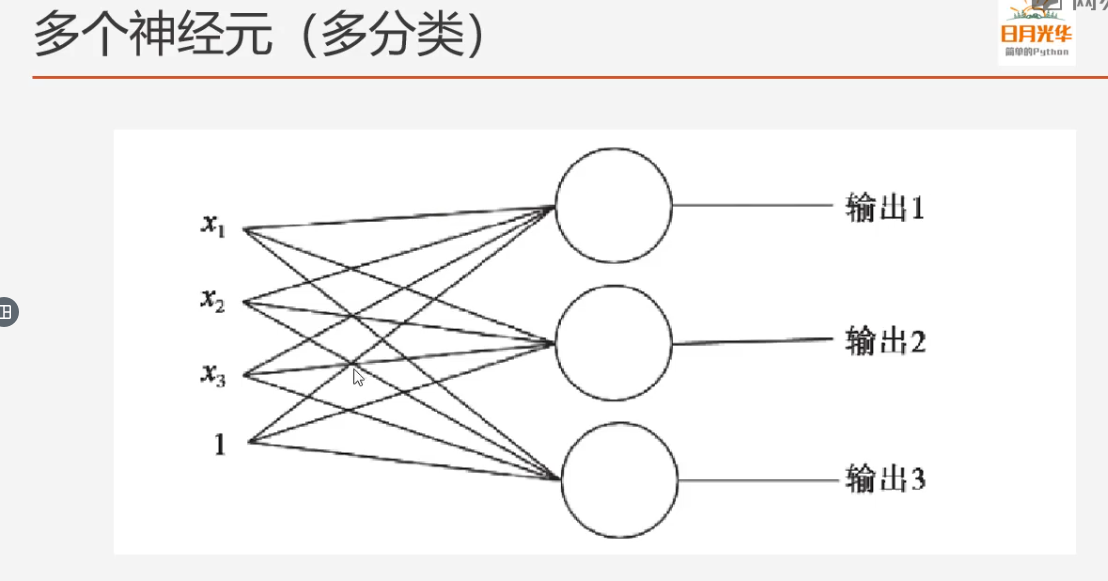展开全文• 答案是，不可以每个人都知道神经网络中的偏置（bias）是什么，而且从人类实现第一个感知器开始，每个人都知道神经元需要添加偏置项。但你是否考虑过我们为什么要使用偏置项呢?就我而言，直到不久前我才弄清楚这个...
• 神经元到神经网络、从神经网络到...神经网络（Neural Network）是从人类大脑神经元的研究中获得灵感，模拟人脑神经元的功能结构，来完成认知任务的一类机器学习算法。还有一类机器学习算法，则不局限于神经元...
从神经元到神经网络、从神经网络到深度学习：神经网络、深度学习、神经元、神经元模型、感知机、感知机困境、深度网络

目录
从神经元到神经网络、从神经网络到深度学习

神经网络：
展开全文算法 网络 深度学习 机器学习
• 从本章开始，将进入神经网络的学习，首先介绍激活函数，因为它是连接感知机和神经网络的桥梁。如果读者认知阅读了本专题知识，相信你必有收获。 感知机数学表达式的简化 前面我们介绍了用感知机接收两个输入信号的...深度学习 python 激活函数
• 作为开始，我介绍一种被称为感知机(perceptron)的人工神经元感知机可以使在1950年到1960年左右由Frank Rosenblatt提出的。感知机的想法是建立在Warren McCulloch Walter Pitts工作的基础上的。今天其他人工神经...人工智能 图像处理
• 神经网络与深度学习方面的一些知识，是一直想做的事情。但本人比较懒惰，有点时间想玩点游戏呀什么的，一直拖到现在。 也由于现在已经快当爸了，心也沉了下来，才能去梳理一些东西。本文会深入检出的去看神经网络...深度学习、神经网络 neuroph
• 一只果蝇大约只有10万个神经元，但是它却可以完成飞翔、觅食等相当复杂的任务。 大脑的排除干扰能力也非常强大，比如说你可以在喧闹的人群中听到别人在喊你的名字。 也就是说 ，大脑在受到干扰，或许接收到的信息以...深度学习 激活函数
• 让我们对感知机神经元作进一步简化， 这样的条件描述感知机的方式是繁琐的，我们可以对其进行两种形式的修改来简化它。第一个变化是将 作为卷积表示， ，这里w与x分别代表权重输入向量。第二个改变是将阈值移动到...人工智能 图像处理
• *激活函数*——连接感知机和神经网络的桥梁 *朴素感知机*——单层网络，激活函数使用阶跃函数 *多层感知机*——神经网络，使用sigmoid函数等平滑的激活函数的多层网络 一、 1.激活函数 a. si...机器学习 深度学习
• M-P模型1943年，McCulloch and Pitts抽象出了“M-P神经元模型”，神经元接受到来自nn个其他神经元传递过来的输入信号，通过带权重的值连接传递，神经元接受到的总输入值与神经元的阈值进行比较，通过激活函数...机器学习 多层网络
• 文章目录神经元单层感知机激活函数多输出感知机单层感知机的几何意义 神经网络的起源是人们力图寻找或者构建一种结构来模拟人脑神经元的功能，并最终达到模拟人脑进行工作甚至达到仿真人的级别。实际上现行的神经...机器学习 线性代数 人工智能
• 今天，使用其他人工神经元模型更为普遍———在这本书中，以及更多现代的神经网络工作中，主要使用的是一种叫做S型神经元神经元模型。 感知器是如何工作的呢？一个感知器接受几个二进制输入，并产生一个二进制输....
• 今天我要分享的是神经元模型以及感知机，并且介绍神经元实现简单的与、或 、非运算 神经元模型 先来看看人体的神经元模型（图源自网络，如有侵权请评论，马上删除） 当左边的神经元接收到皮肤等感受器官的电信号...机器学习
• 参考文章： 一看就懂的感知机算法PLA（基础概念） ...由McCullochPitts于1943年发表，简单模拟了神经元的反应流程，包括： 多个带有权重的输入wi×xiw_i×x_iwi​×xi​，相当于「突触」 xix_ixi​是输入值，...机器学习
• 二十世纪四十年代 M-P 神经元模型、Hebb 学习律出现后，五十年代出现了以感知机、Adaline 为代表的一系列成果。后来因为单层神经网络无法解决非线性问题，而多层网络的训练算法尚看不到希望，神经网络研究进入“冰河...机器学习
• 3.线性阈值神经元特征：输入输出都是二值的，每个神经元都有一个固定的阈值，每个神经元都从带有权重的激活突触接受输入信息，抑制突触对任意激活突触有绝对否决权，每次汇总带权突触的，如果大于阈值而且不存在...深度学习 机器学习 神经网络
• 感知机–多层感知机 MLP–神经网络 感知机也叫感知器 简单感知机是一个单层神经网络。它使用阈值激活函数 叠加了多层的感知机也称为多层感知机(multi...神经网络中的神经元的祖先是感知器 前馈网络 数据进入输入层, ...
• 3.2多层感知机和神经网络 3.2.1定义： 多层感知机是在单层神经网络上引入一个或多个隐藏层，即输入层，隐藏层，输出层。 3.2.2网络退化问题 网络退化就是多层感知机会退化成单层 激活函数可以防止退化。 多层感知机...
• DL之Perceptron：Perceptron感知器(感知机/多层感知机/人工神经元)的简介、原理、案例应用(相关配图)之详细攻略 目录 Perceptron的简介、原理 多层感知机 实现代码 案例应用 相关文章DL之AF：机器...
• 深度篇——神经网络(一) 神经元感知机 深度篇——神经网络(二) ANN与DNN 结构常用激活函数 深度篇——神经网络(三) 网络拓扑与训练神经网络 深度篇——神经网络(四) 细说 调优神经网络 深度篇——神经网络...深度学习
• 1.感知器  一个感知器接受几个二进制输入,x 1 , x 2 , . . .,并产生一个二进制输出:   示例中的感知器有三个输入,x 1 , x 2 , x 3，引入...神经元的输出,0 或者 1,则由分配权重后的总和∑jwjxj is小于或者大于一深度学习
• 1. 线性感知机 例如一个房屋价格预测问题。输入 x 是房屋面积大小，输出 y 是房屋的价格。如果要预测价格与面积的关系，最简单的一种模型就是 y 与 x 近似线性相关。 如上图所示，红色圆圈表示真实样本的价格与面积......